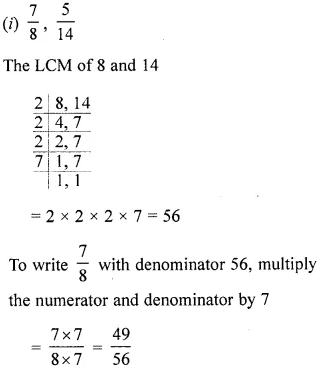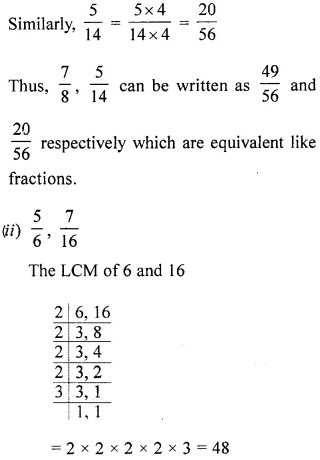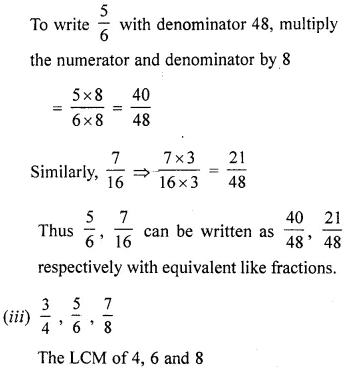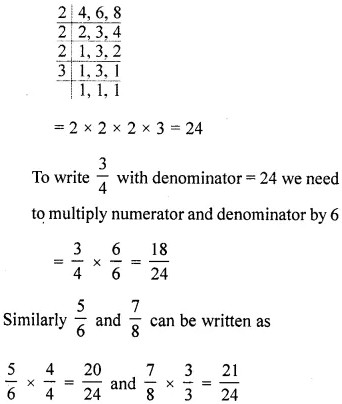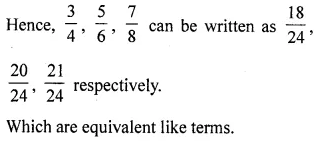# ML Aggarwal Class 6 Solutions for ICSE Maths Chapter 6 Fractions Ex 6.3

## ML Aggarwal Class 6 Solutions for ICSE Maths Chapter 6 Fractions Ex 6.3

Question 1.
State which of the following fractions are proper, improper or mixed: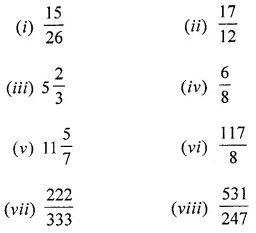Solution: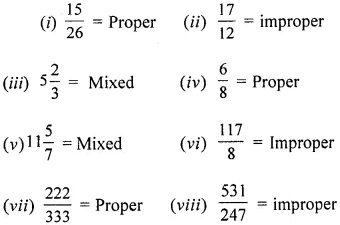Question 2.
Convert the following improper fractions into mixed numbers:Solution: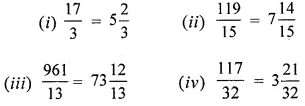Question 3.
Convert the following mixed number into improper fractions:Solution:Question 4.
Write the fractions representing the shaded regions. Are all these fractions equivalent?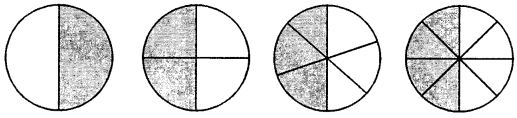Solution: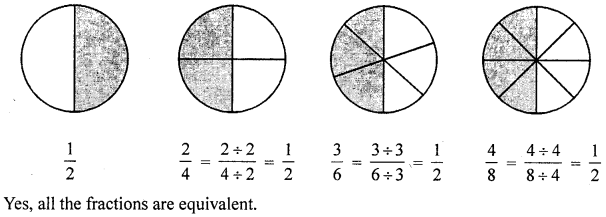Question 5.
Write the fractions representing the shaded regions and pair up the equivalent fractions from each row: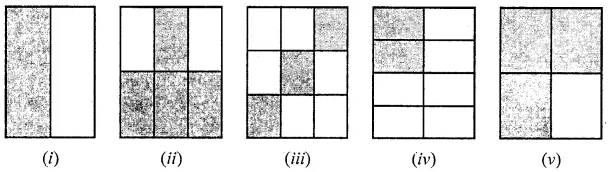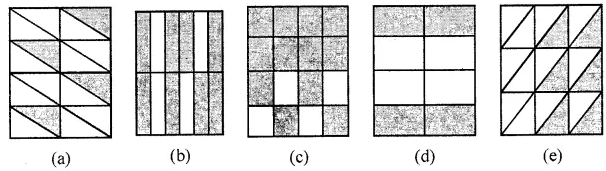Solution: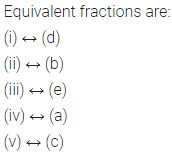Question 6.
(i) Find the equivalent fraction of $$\frac{15}{35}$$ with denominator 7.
(ii) Find the equivalent fraction of $$\frac{2}{9}$$ with denominator 63.
Solution:Question 7.
Find the equivalent fraction of $$\frac{3}{5}$$ having
(i) denominator 30
(ii) numerator 27.
Solution:Question 8.
Replace ‘…..’ in each of the following by the correct number.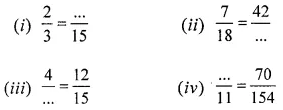Solution:Question 9.
Check whether the given pairs of fractions are equivalent: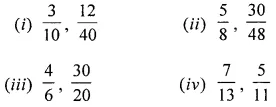Solution: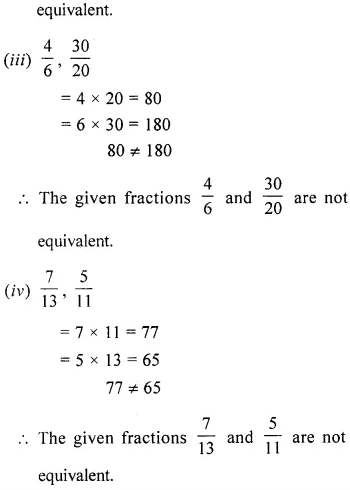Question 10.
Reduce the following fractions to simplest form: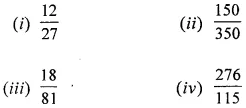Solution: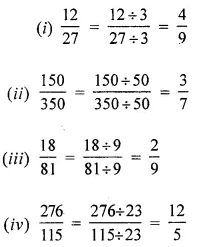Question 11.
Convert the following fractions into equivalent like fractions:Solution: